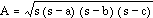# Heron’s Area

In the world of triangles with sides of integral length, the Heron triangle has an integral area. Heron triangles are named after Heron of Alexandria, a Greek scientist who lived during the first century A.D. and is credited with the ingenious formula relating the area of a triangle to the length of its sides:.
Here a, b, c stand for the length of the sides and s = (a + b + c) / 2. Note that s stands for half of the perimeter, or the semi-perimeter. Primitive Heron triangles have side measurements whose greatest common divisor is one.

1. List all the different primitive Heron triangles with area less than or equal to 100.
2. How many different primitive Heron triangles are there with sides less than or equal to 25? 50? 100?
3. Which of the triangles in questions 1 and 2 is closest to be an equilateral?
4. Comment on the truth of the following statements:
• The length of a side of a Heron triangle is even.
• The length of a side of a Heron triangle is a multiple of 3.
• The length of a side of a Heron triangle is a multiple of 5.
• The area of a Heron triangle is a multiple of 6.
5. It is said that every Heron triangle has at least one altitude of integral length. Is this true?